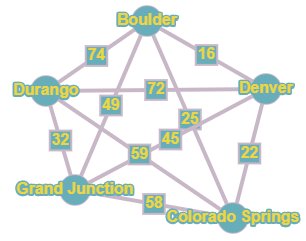# Travel The prices for traveling between five cities in Colorado by bus are given in the table below. Represent the travel costs between cities using a weighted graph. Use the greedy algorithm to find a low-cost route that starts and ends in Boulder and visits each city.### Mathematical Excursions (MindTap C...

4th Edition
Richard N. Aufmann + 3 others
Publisher: Cengage Learning
ISBN: 9781305965584

#### Solutions

Chapter
Section### Mathematical Excursions (MindTap C...

4th Edition
Richard N. Aufmann + 3 others
Publisher: Cengage Learning
ISBN: 9781305965584
Chapter 5.2, Problem 24ES
Textbook Problem
1 views

## Travel The prices for traveling between five cities in Colorado by bus are given in the table below. Represent the travel costs between cities using a weighted graph. Use the greedy algorithm to find a low-cost route that starts and ends in Boulder and visits each city.To determine

To represent the travel costs between the cities by a weighted graph and to find a low-cost route for the tour using the greedy algorithm.

### Explanation of Solution

Given information:

Given, the prices for travelling between five cities in Colorado by bus are given in the table below:

The travel starts and ends in Boulder and visits each city.

Calculation:

Given, there are five cities to travel which starts and ends at Boulder.

Hence five vertices corresponding to the five cities are marked with respective names.

And then making the complete graph by marking every possible edge with every edge marked with the weight represented in the table between the cities.

The airfare between Boulder and Denver is \$16. Hence the edge drawn between them has a weight of 16.

Similarly marking all the edges with respective weights, the graph is given as:

To use the greedy algorithm, the graph must be Hamiltonian.

(Since, the tourist needs to visit each city only once)

A path in a Hamiltonian graph is said to be a Hamiltonian Circuit if it begins and ends at the same vertex and passes through each vertex of a graph exactly once.

The greedy algorithm states to travel along a connected edge that has the smallest weight that is not yet visited. This process continues till all the vertices are visited and returned to the starting vertex.

Here, the vertex representing Boulder is selected.

Vertex representing Boulder is of degree 4 and the weights of the connected edges are 16, 25, 49, 74

Hence, as per the Greedy Algorithm the edge with weight 16 is selected i.e. path reaches vertex representing Denver.

Vertex representing Denver is of degree 4 and the weights of the connected edges are 16, 22, 45, 72

The least of the weights is 16 but it leads to vertex representing Boulder which is already visited...

### Still sussing out bartleby?

Check out a sample textbook solution.

See a sample solution

#### The Solution to Your Study Problems

Bartleby provides explanations to thousands of textbook problems written by our experts, many with advanced degrees!

Get Started

Find more solutions based on key concepts
In Exercises 5762, sketch the straight line defined by the given linear equation by finding the x- and y-interc...

Applied Calculus for the Managerial, Life, and Social Sciences: A Brief Approach

Sketch the graphs of the equations in Exercises 512. xy=2x3+1

Finite Mathematics and Applied Calculus (MindTap Course List)

Change each fraction to a percent: 34

Elementary Technical Mathematics

Solve the initial-value problem. 16. t3dydt+3t2y=cost, y() = 0

Single Variable Calculus: Early Transcendentals

For f (x) = sinh x, f (x) = a) cosh2 x b) sinh2 x c) tanh x d) sinh x

Study Guide for Stewart's Single Variable Calculus: Early Transcendentals, 8th